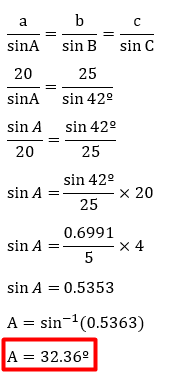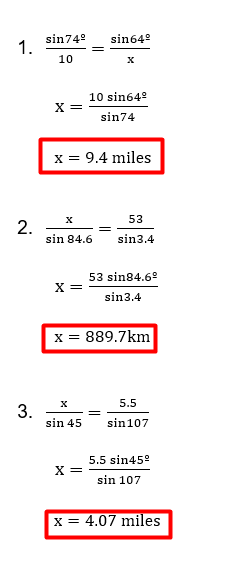# Law of Sines Word Problems worksheet (PDF + with answer key)

The relationship between an oblique triangle’s sides and angles is established by the law of sines (non-right triangle). Important trigonometrical laws used in “solving a triangle” include the laws of sines and cosines. The ratio of a triangle’s side lengths to the sine of each of its opposite angles must be equal in order for the sine rule to apply.

What is the “Law of Sines Word Problems worksheet (PDF)”?

This worksheet will explore some of the Law of Sines Word Problems.

## What is the Law of Sines?

Triangles’ side length to opposite angle ratios is based on the law of sines. For all three sides and diametrically opposed angles, this ratio remains constant. Therefore, using the necessary known information, we can use the sine rule to determine the missing side or angle of any triangle.

This worksheet will help you to understand the topic and, in the sections, below, using examples that have been solved, let’s learn how to calculate sines and how to prove them.

Instructions on how to use the “Law of Sines Word Problems worksheet (PDF).”

Study the concept and examples given and try to solve the given exercises below.

## Conclusion

The ratio of a triangle’s side to its corresponding angle is equal to the circumference of the triangle is known as the Law of Sines.

If you have any inquiries or feedback, please let us know.

## Law of Sines Word Problems worksheet (PDF + with answer key)

Law of Sines Definition

According to the Law of Sines, a triangle’s side and corresponding angle are proportional to the circumference of the triangle.

Law of Sines Formula

• a, b, and c are the lengths of the triangle
• A, B, and C are the angles of the triangle.

This formula can be represented in three different forms given as,

Example:

Given a = 20 units c = 25 units and Angle C = 42º. Find the angle A of the triangle.For example

Two observers, Anna and Boy, sight a helicopter at angles of 44 º and 66 º, respectively. If Anna has located 15 km from the helicopter, find the horizontal distance between Boy and Anna.

## Law of Sines.

1. Fire towers A and B are located 10 miles apart. They use the direction of the other tower as 0 º. Rangers at fire tower A spot fire at 42º, and rangers at fire tower B spot the same fire at 64º. How far from tower A is the fire to the nearest tenth of a mile?1. Tracking stations A and B, are 53 km apart. When the satellite is on one side of the two stations, the angles of elevation at A and B are measured to be 88º and 84.6º, respectively.1. A pilot is flying over a straight-line highway between mileposts A and B. She determines the angles of depression to two mileposts. A and B, which are 5.5 miles apart, are 28º and 45º, respectively. Find the distance of the plane to point A.